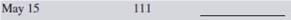# Contemporary Mathematics

Mathematics

## Quiz 10 : Simple Interest and Promissory NotesStudy FlashcardsLooking for Developmental Mathematics Homework Help?

## Quiz 10 :Simple Interest and Promissory Notes

Question TypeWhat is the maturity value of the following loans? Use MV = P (1 + RT ) to find the maturity values.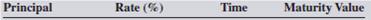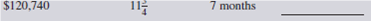Free
Essay

To find the maturity value of a loan of \$120,740 at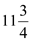% interest for 7 months, use the maturity value formula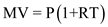where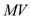is the maturity value,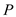is the principal R is the rate of interest and T is the time factor.
Here the rate% is converted to a decimal 0.1175 and the time factor, expressed as a fraction of a year,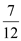.
Now substituting 120,740 for P , 0.1175 for R andfor T in the simple interest formula givesHence the maturity value of the loan is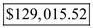.

Tags
Choose question tagInterest calculated solely on the principal amount borrowed is known as __________ interest, while interest calculated at regular intervals on the principal and previously earned interest is known as __________ interest.
Free
Essay

Interest is the price or rental fee charged by a lender to a business or an individual for the use of money. Interest calculated solely on the principal amount borrowed is known as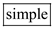interest, while interest calculated at regular intervals on the principal and the previously earned interest is known as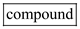interest.
Simple interest loans are usually made for short periods of time, such as a few days, weeks or months, whereas compound interest loans are generally for time periods of a year or longer.

Tags
Choose question tagMaturity value is the total payback of principal and interest of a loan. List the two formulas for calculating maturity value.
Free
Essay

When the time period of a loan is over, the loan is said to be mature. At that time, the borrower repays the original principal plus the interest. Maturity value is the total payback of principal and interest of a loan. Once the interest has been calculated, the maturity value can be found by using the formula: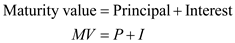Maturity value can also be calculated directly without first calculating the interest by using the following formula: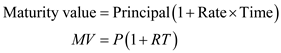Tags
Choose question tagThe interest calculation method that uses 360 days as the time factor denominator is known as __________ interest.
Essay
Tags
Choose question tagUsing the ordinary interest method (360 days), find the amount of interest on the following loans.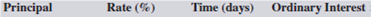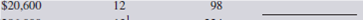Essay
Tags
Choose question tagThe interest calculation method that uses 365 days (366 in leap year) as the time factor denominator is known as __________ interest.
Essay
Tags
Choose question tagThe first day of a loan is known as the __________ date; the last day of a loan is known as the __________ date.
Essay
Tags
Choose question tagWhat is the maturity value of the following loans? Use MV = P ( 1 + RT ) to find the maturity values.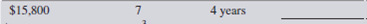Essay
Tags
Choose question tagThe U.S. rule states that when a partial payment is made on a loan, the payment is first used to pay offthe accumulated __________ to date and the balance is used to reduce the __________.
Essay
Tags
Choose question tagUsing the exact interest method (365 days), find the amount of interest on the following loans.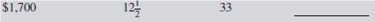Essay
Tags
Choose question tagWrite the formula for calculating simple interest.
Essay
Tags
Choose question tagList the three factors that determine the amount of interest charged on a loan.
Essay
Tags
Choose question tagUsing the ordinary interest method (360 days), find the amount of interest on the following loans.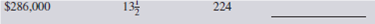Essay
Tags
Choose question tagFrom the following information, determine the number of days of each loan.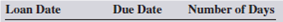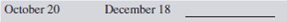Essay
Tags
Choose question tagThe price or rental fee charged by a lender to a borrower for the use of money is known as __________.
Essay
Tags
Choose question tagFrom the following information, determine the maturity date of each loan.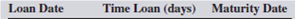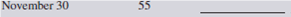Essay
Tags
Choose question tagUsing the exact interest method (365 days), find the amount of interest on the following loans.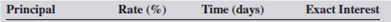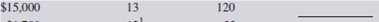Essay
Tags
Choose question tagFrom the following information, determine the number of days of each loan.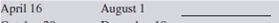Essay
Tags
Choose question tagWhen solving the simple interest formula for principal, rate, or time, the __________ is always the numerator.
EssayFrom the following information, determine the maturity date of each loan.